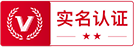##用户名 Email 自动登录 找回密码 密码 立即注册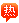# 10 10 10 10=只能用符号连接分别等于1,2,3,4…

2020-7-18 15:42| 发布者: admin| 查看: 10| 评论: 0

### 二蛋天王的回答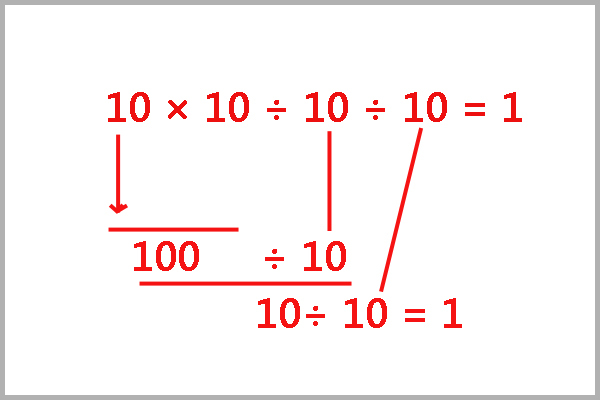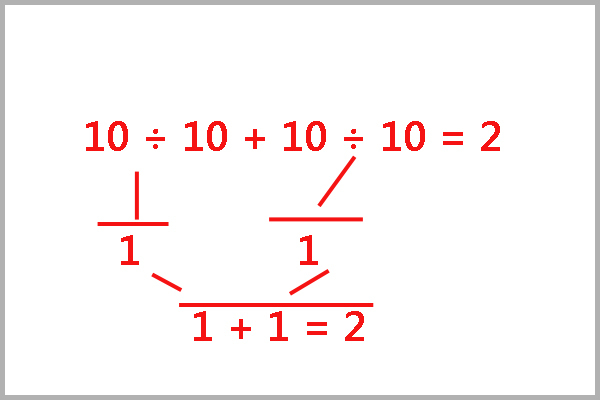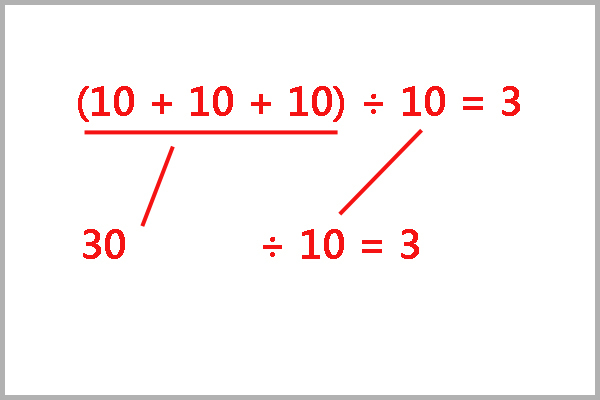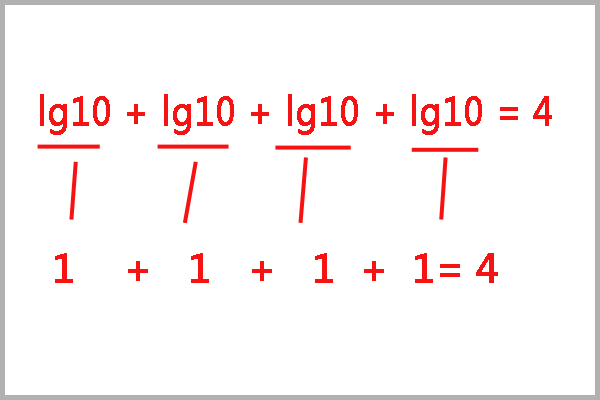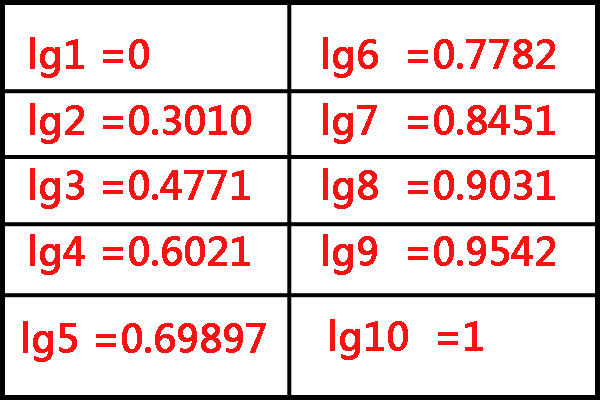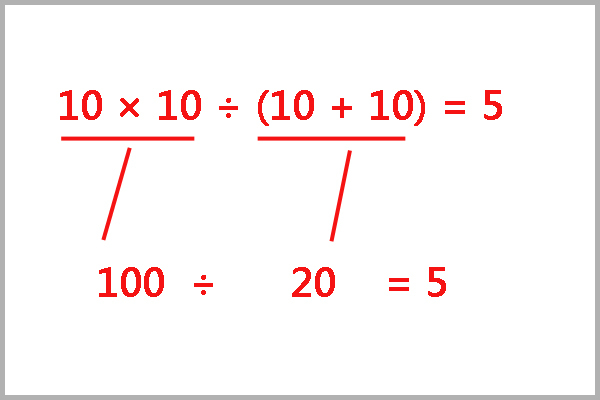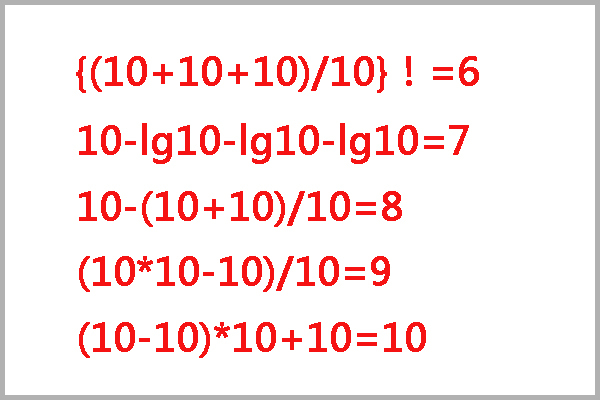### 匿名用户的回答

10÷10×10÷10=1，
10÷10+10÷10=2，
（10+10+10）÷10=3，
lg10+lg10+lg10+lg10=1+1+1+1=4，

lg是10的对数函数，lg10=1，

### _夏布衫的回答

10*10/10/10=1
10/10-10/10=2
(10+10+10)/10=3
lg10+lg10+lg10+lg10=4
10/{(10+10)/10}=5
{(10+10+10)/10}！=6
10-lg10-lg10-lg10=7
10-(10+10)/10=8
(10*10-10)/10=9
(10-10)*10+10=10

### 匿名用户的回答

10 ÷ 10 ÷ 10 ÷ 10=1
10 ÷ 10 + 10 ÷ 10=2
lg10+lg10+10÷10=3
lg10+lg10+lg10+lg10=4
（10x10）÷(10+10)=5
（lg10+lg10+10÷10）=6
10 - lg（10x10x10）=7
10 x lg10-lg（10x10）=8
10x10÷10-lg10=9
（10x10）÷10÷lg10=10

### 最新评论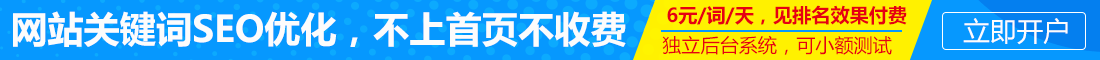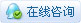|广告服务|网站出售|投稿要求|禁言标准|版权说明|免责声明|手机版|小黑屋|推扬网 ( 粤ICP备18134897号 )|网站地图

GMT+8, 2021-1-20 02:31 , Processed in 0.460857 second(s), 29 queries .